Area of similar triangles

Chapter 6 Class 10 Triangles
Concept wise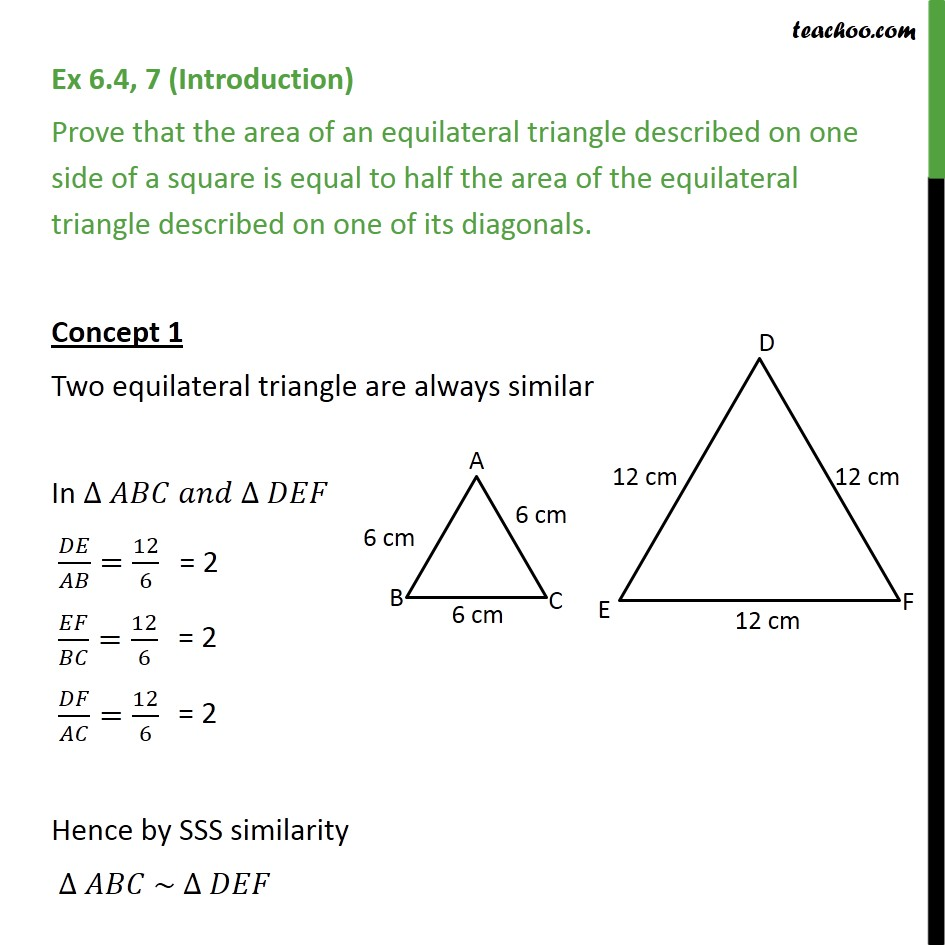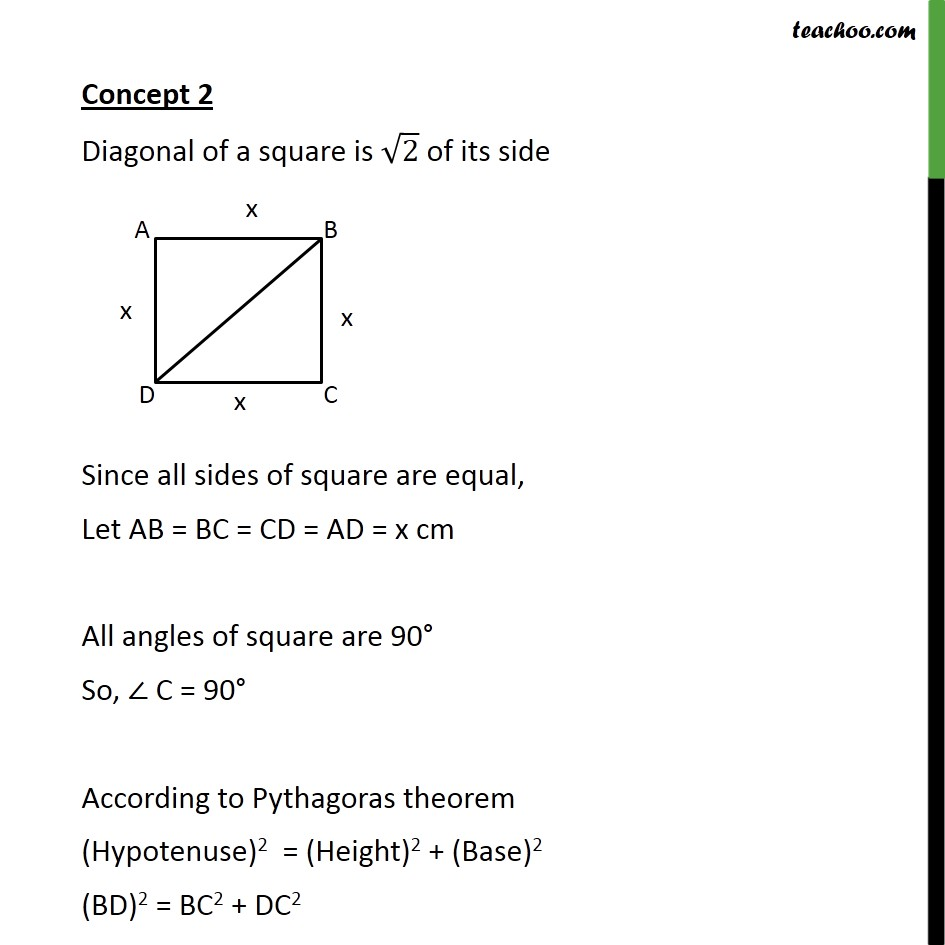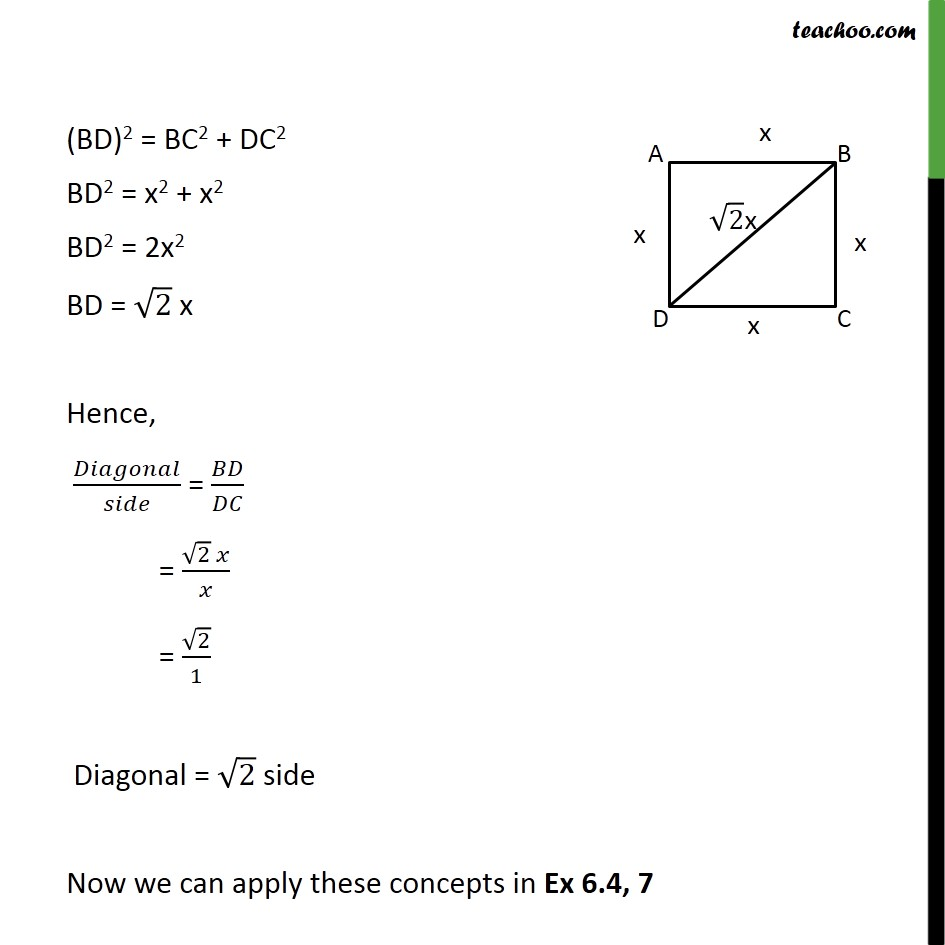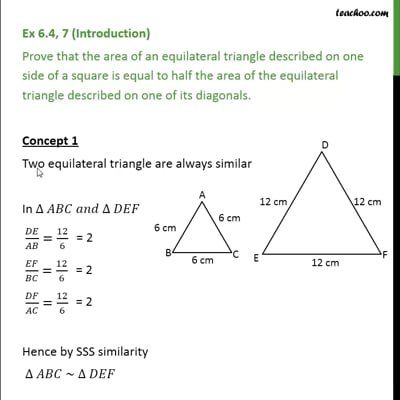This video is only available for Teachoo black users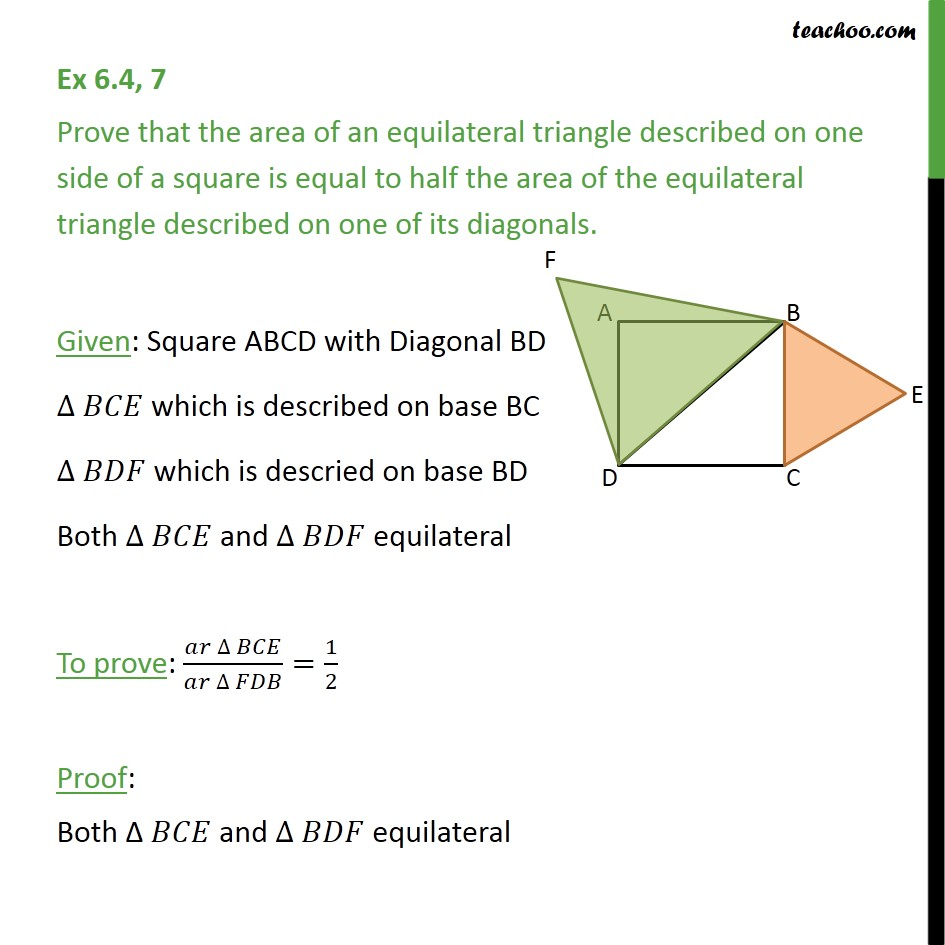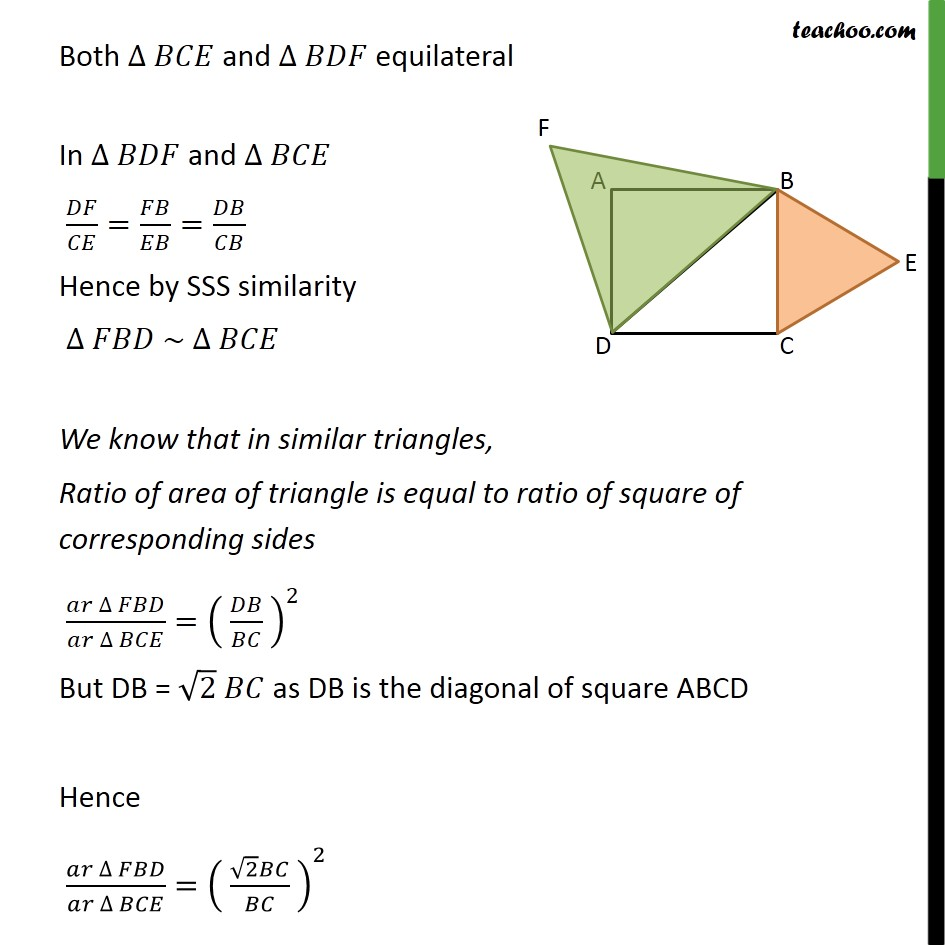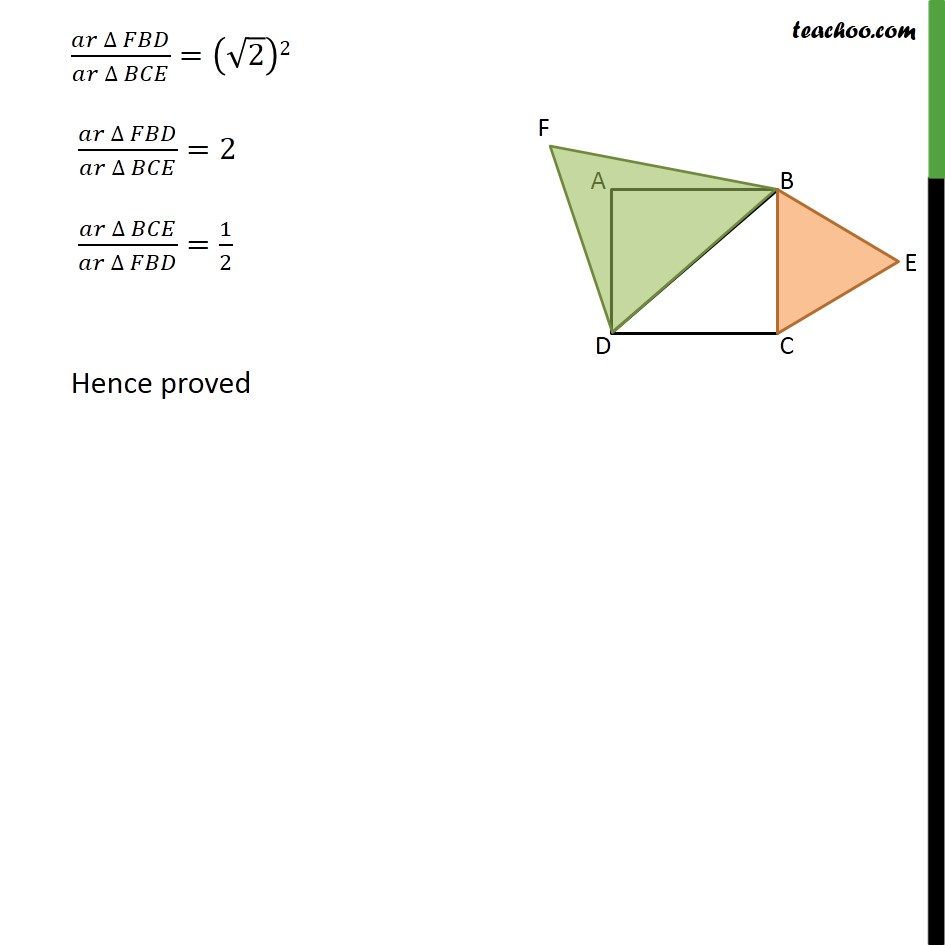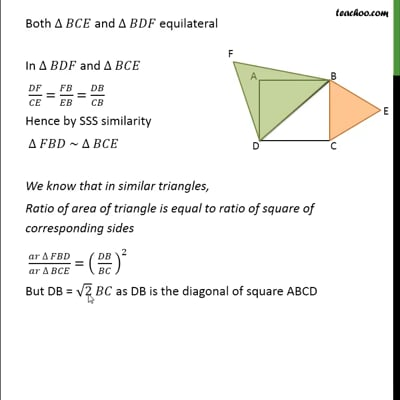This video is only available for Teachoo black users

Solve all your doubts with Teachoo Black (new monthly pack available now!)

### Transcript

Ex 6.4, 7 (Introduction) Prove that the area of an equilateral triangle described on one side of a square is equal to half the area of the equilateral triangle described on one of its diagonals. Concept 1 Two equilateral triangle are always similar In ∆ 𝐴𝐵𝐶 𝑎𝑛𝑑 ∆ 𝐷𝐸𝐹 𝐷𝐸/𝐴𝐵=12/6 𝐸𝐹/𝐵𝐶=12/6 𝐷𝐹/𝐴𝐶=12/6 Hence by SSS similarity ∆ 𝐴𝐵𝐶 ~ ∆ 𝐷𝐸𝐹 Concept 2 Diagonal of a square is √2 of its side Since all sides of square are equal, Let AB = BC = CD = AD = x cm All angles of square are 90° So, ∠ C = 90° According to Pythagoras theorem (Hypotenuse)2 = (Height)2 + (Base)2 (BD)2 = BC2 + DC2 (BD)2 = BC2 + DC2 BD2 = x2 + x2 BD2 = 2x2 BD = √2 x Hence, 𝐷𝑖𝑎𝑔𝑜𝑛𝑎𝑙/𝑠𝑖𝑑𝑒 = 𝐵𝐷/𝐷𝐶 = (√2 𝑥)/𝑥 = √2/1 Diagonal = √2 side Now we can apply these concepts in Ex 6.4, 7 Ex 6.4, 7 Prove that the area of an equilateral triangle described on one side of a square is equal to half the area of the equilateral triangle described on one of its diagonals. Given: Square ABCD with Diagonal BD ∆ 𝐵𝐶𝐸 which is described on base BC ∆ 𝐵𝐷𝐹 which is descried on base BD Both ∆ 𝐵𝐶𝐸 and ∆ 𝐵𝐷𝐹 equilateral To prove: (𝑎𝑟 ∆ 𝐵𝐶𝐸)/(𝑎𝑟 ∆ 𝐹𝐷𝐵)=1/2 Proof: Both Δ 𝐵𝐶𝐸 and ∆ 𝐵𝐷𝐹 equilateral Both ∆ 𝐵𝐶𝐸 and ∆ 𝐵𝐷𝐹 equilateral In ∆ 𝐵𝐷𝐹 and ∆ 𝐵𝐶𝐸 𝐷𝐹/𝐶𝐸=𝐹𝐵/𝐸𝐵=𝐷𝐵/𝐶𝐵 Hence by SSS similarity ∆ 𝐹𝐵𝐷 ~ ∆ 𝐵𝐶𝐸 We know that in similar triangles, Ratio of area of triangle is equal to ratio of square of corresponding sides (𝑎𝑟 ∆ 𝐹𝐵𝐷)/(𝑎𝑟 ∆ 𝐵𝐶𝐸)=( 𝐷𝐵/𝐵𝐶 )^2 But DB = √2 𝐵𝐶 as DB is the diagonal of square ABCD Hence (𝑎𝑟 ∆ 𝐹𝐵𝐷)/(𝑎𝑟 ∆ 𝐵𝐶𝐸)=( (√2 𝐵𝐶)/𝐵𝐶 )^2 (𝑎𝑟 ∆ 𝐹𝐵𝐷)/(𝑎𝑟 ∆ 𝐵𝐶𝐸)=(√2)2 (𝑎𝑟 ∆ 𝐹𝐵𝐷)/(𝑎𝑟 ∆ 𝐵𝐶𝐸)=2 (𝑎𝑟 ∆ 𝐵𝐶𝐸)/(𝑎𝑟 ∆ 𝐹𝐵𝐷)=1/2 Hence proved